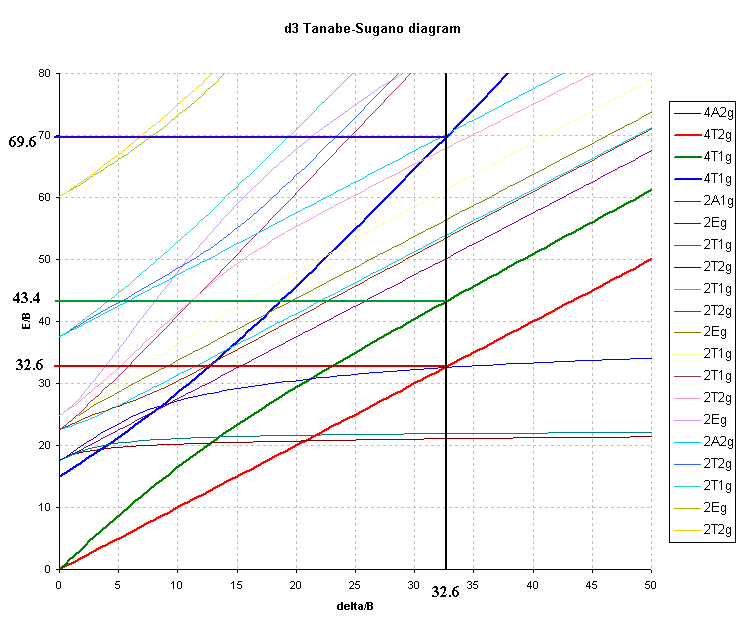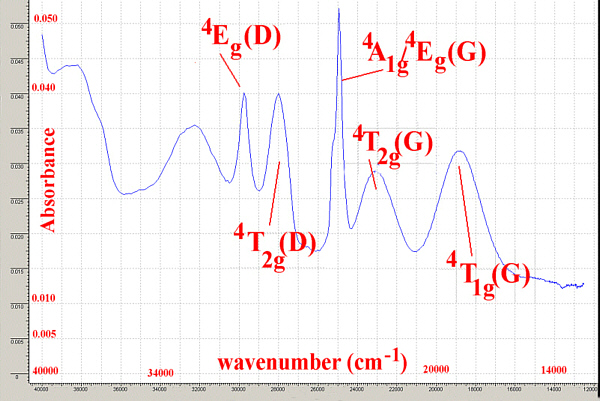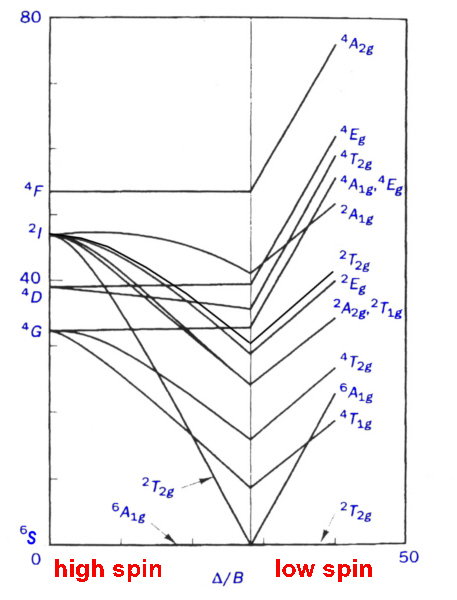## Interpretation of the spectra of first-row transition metal complexes using Tanabe-Sugano diagrams

For ALL octahedral complexes, except high spin d5, simple CFT would predict that only 1 band should appear in the electronic spectrum and that the energy of this band should correspond to the absorption of energy equivalent to Δ. In practice, ignoring spin-forbidden lines, this is only observed for those ions with D free ion ground terms i.e., d1, d9 as well as d4, d6.

The observation of 2 or 3 peaks in the electronic spectra of d2, d3, d7 and d8 high spin octahedral complexes requires further treatment involving electron-electron interactions. Using the Russell-Saunders (LS) coupling scheme, these free ion configurations give rise to F free ion ground states which in octahedral and tetrahedral fields are split into terms designated by the symbols A2(g), T2(g) and T1(g).

To derive the energies of these terms and the transition energies between them is beyond the needs of introductory level courses and is not covered in general textbooks[10,11]. A listing of some of them is given here as an Appendix. What is necessary is an understanding of how to use the diagrams, created to display the energy levels, in the interpretation of spectra.

In the laboratory component of the course we will measure the absorption spectra of some typical chromium(III) complexes and calculate the spectrochemical splitting factor, Δ. This corresponds to the energy found from the first transition below and as shown in Table 1 is generally between 15,000 cm-1 (for weak field complexes) and 27,000 cm-1 (for strong field complexes).

For the d3 octahedral case, 3 peaks can be predicted and these would correspond to the following transitions and energies:

1. 4T2g4A2g ĀĀĀĀĀĀĀĀĀĀ transition energy = Δ
2. 4T1g(F) ← 4A2g ĀĀĀĀĀ transition energy = 9/5 * Δ - C.I.
3. 4T1g(P) ← 4A2g ĀĀĀĀĀ transition energy = 6/5 * Δ + 15B' + C.I.

where C.I. is the configuration interaction arising from the "non-crossing rule".

 Complex ν1 ν2 ν3 ν2/ ν1 ν1/ ν2 Δ/B Ref Cr3+ in emerald 16260 23700 37740 1.46 0.686 20.4 13 K2NaCrF6 16050 23260 35460 1.45 0.690 21.4 13 [Cr(H2O)6]3+ 17000 24000 37500 1.41 0.708 24.5 This work Chrome alum 17400 24500 37800 1.36 0.710 29.2 4 [Cr(C2O4)3]3- 17544 23866 ? 1.37 0.735 28.0 This work [Cr(NCS)6]3- 17800 23800 ? 1.34 0.748 31.1 4 [Cr(acac)3] 17860 23800 ? 1.33 0.752 31.5 This work [Cr(NH3)6]3+ 21550 28500 ? 1.32 0.756 32.6 4 [Cr(en)3]3+ 21600 28500 ? 1.32 0.758 33.0 4 [Cr(CN)6]3- 26700 32200 ? 1.21 0.829 52.4 4

For octahedral Ni(II) complexes the transitions would be:

1. 3T2g3A2g ĀĀĀĀĀĀĀĀĀĀ transition energy = Δ
2. 3T1g(F) ← 3A2g ĀĀĀĀĀ transition energy = 9/5 * Δ - C.I.
3. 3T1g(P) ← 3A2g ĀĀĀĀĀ transition energy = 6/5 * Δ + 15B' + C.I.

where C.I. again is the configuration interaction and as before the first transition corresponds exactly to Δ.

For M(II) the size of Δ is much less than for M(III) and typical values for Ni(II) are 6500 to 13000 cm-1 as shown in Table 2.

 Complex ν1 ν2 ν3 ν1 ν1/ ν2 Δ/B Ref NiBr2 6800 11800 20600 1.74 0.576 5 13 [Ni(H2O)6]2+ 8500 13800 25300 1.62 0.616 11.6 13 [Ni(gly)3]- 10100 16600 27600 1.64 0.608 10.6 13 [Ni(NH3)6]2+ 10750 17500 28200 1.63 0.614 11.2 13 [Ni(en)3]2+ 11200 18350 29000 1.64 0.610 10.6 3 [Ni(bipy)3]2+ 12650 19200 ? 1.52 0.659 17 3

For d2 octahedral complexes, few examples have been published. One such is V3+ doped in Al2O3 where the vanadium ion is generally regarded as octahedral, Table 3.

 Complex ν1 ν2 ν3 ν2/ ν1 ν1/ν2 Δ/B Ref V3+ in Al2O3 17400 25200 34500 1.448 0.6906 30.90 13 [VCl3(MeCN)3] 14400 21400 ? 1.486 0.6729 28.68 4 K3[VF6] 14800 23250 ? 1.571 0.6365 24.78 4

Interpretation of the spectrum highlights the difficulty of using the right-hand side of the Orgel diagram as previously noted. For d2 cases where none of the transitions correspond exactly to Δ often only 2 of the 3 transitions are clearly observed and hence the calculations will have three unknowns (Δ, B and C.I.) but only 2 energies to use in the the analysis.

The first transition can be unambiguously assigned as:

3T2g3T1g ĀĀĀĀĀĀĀĀĀ transition energy = 4/5 * Δ + C.I.

But, depending on the size of the ligand field ( Δ) the second transition may be due to:

3A2g3T1g ĀĀĀĀĀĀĀĀĀ transition energy = 9/5 * Δ + C.I.

for a weak field or

3T1g(P) ← 3T1g ĀĀĀĀĀ transition energy = 3/5 * Δ + 15B' + 2 * C.I.

for a strong field.

The transition energies of these terms are clearly different and it is often necessary to calculate (or estimate) values of B, Δ and C.I. for both arrangements and then evaluate the answers to see which fits better.

The difference between the 3A2g and the 3T2g (F) lines should give Δ. In this case Δ is equal to either:
25200 - 17400 = 7800 cm-1
or 34500 - 17400 = 17100 cm-1.
Given that we expect Δ to be greater than 15000 cm-1 then we must interpret the second transition as to the 3T2g(P) and the third to 3A2g. Further evaluation of the expressions then gives C.I. as 3720 cm-1 and B' as 567 cm-1.

Solving the equations like this for the three unknowns can ONLY be done if the three transitions are observed. When only two transitions are observed, a series of equations have been determined that can be used to calculate both B and Δ. This approach still requires some evaluation of the numbers to ensure a valid fit. For this reason, Tanabe-Sugano diagrams become a better method for interpreting spectra of d2 octahedral complexes.

### Using Tanabe-Sugano diagrams

The use of Orgel diagrams allows a qualitative description of the spin-allowed electronic transitions expected for states derived from D and F ground terms. Only 2 diagrams are needed for high spin d2-d9 and both tetrahedral and octahedral ions are covered.

Tanabe-Sugano diagrams were developed in the 1950's to give a semi-quantitative approach and include both high and low spin ions and not only the spin-allowed transitions are shown but the spin-forbidden transitions are displayed as well.

At first glance they can appear quite daunting, but in practice they are much easier to use for interpreting spectra and provide much more information. The obvious differences are the presence of the additional lines and that the ground state is shown as the base line along the X axis rather than as a straight line or curve originating from the Y axis.

On the X axis Δ/B' is plotted while on the Y axis E/B' is plotted, where B' is the modified Racah B parameter that exists in the complex.

A separate diagram is needed for each electronic configuration d2-d9 and for the d4-d7 cases both the high spin and low spin electronic configurations are shown. The high spin is on the left-hand-side of the vertical line on the diagram.

For the d2 case where it is difficult to use an Orgel diagram, the TS diagram is shown below. The ground state is 3T1g which is plotted along the base line.
Note that the transitions that occur are dependent on the sizes of Δ and B and the A2g term may be either higher or lower than the T1g (P) term (depending on whether Δ/B' is greater than about 15).

For the V(III) aqua ion, transitions are observed at 17,200 and 25,600 cm-1 which are assigned to the 3T2g ← 3T1g and 3T1g(P) ← 3T1g (F) respectively.
Interpretation requires taking the ratio of these frequencies and then finding the position on the diagram where the height of the 3T1g(P) / 3T2g exactly matches that ratio.

For a ratio of 1.49, this is found on the diagram below at Δ/B' of 28.0.Reading off the position on the Y axis for the three spin-allowed lines gives E/B' values of 25.9, 38.6 and 53 (3T2g, 3T1g and 3A2g)

To determine the value of Δ and B' is now relatively straightforward since from the first transition energy of 17,200 cm-1 and the value of E/B' of 25.9 we can equate B' as:
B' = 17,200/ 25.9 or B' = 665 cm-1
Alternatively from the second transition energy of 25,600 cm-1 and the value of E/B' of 38.6 we can equate B' as:
B' = 25,600/ 38.6 or B' = 663 cm-1 which is in excellent agreement with the value found from the first transition.

The value of Δ can then be determined from the Δ/B' ratio of 28.0 and the value just calculated for B' of 665 cm-1.
This gives Δ as 28.0 x 665 = 18,600 cm-1

The transition 3A2g ← 3T1g would be predicted to occur at 53 x 665 that is 35,245 cm-1 (or 284 nm) which is in the UV region and not observed. (Possibly obscured by charge transfer bands).

The values of Δ and B' can be compared to similar V(III) complexes and it should be noted that in general for M(III) ions the Δ value is often about 3/2 times the value expected for M(II) ions.

The free ion value of B for a V(III) ion is 860 cm-1 and the reduction of this value noted for the observed B' is a measure of what is described as the Nephelauxetic Effect.

### The Nephelauxetic Effect

The Racah repulsion parameters for a metal complex vary as the ligand is changed
• As the complex becomes more covalent the electrons are to some extent spread over the ligands so the electron-electron repulsion is reduced
• This reduction in repulsion as covalency increases is called the nephelauxetic effect (literally "cloud expanding")
A nephelauxetic series can be set up based on the variation of the Racah parameter.
A large reduction in B (free ion) indicates a strong Nephelauxetic Effect.

{B(free ion)- B'(Complex)} / B(free ion)

The Nephelauxetic Series is given by:

```  F- < H2O < urea < NH3 < en ~ C2O42- < NCS- < Cl- ~ CN- < Br- < S2- ~ I-
```
This series is consistent with fluoride complexes being the most ionic and giving a small reduction in B while covalently bonded ligands such as I- give a large reduction of B.

N.B. The order of the nephelauxetic series is NOT the same as the spectrochemical series as one is an indication of B and the other of Δ

As an example of a Cr(III) complex, using the observed peaks found for [Cr(NH3)6]3+ in Table 1 above, namely ν1 = 21550 cm-1 and ν2 = 28500 cm-1 the ratio of ν21 = 1.32.
The value of Δ is obtained directly from the first transition so Δ/B' is equal to ν1/B' and finding B' is now relatively straightforward since from the first transition energy of 21,550 cm-1 and the value of Δ/B' (ν1/B') of 32.6 we get:
B' = 21,550/ 32.6 or B' = 661 cm-1
The third peak can then be predicted to occur at 69.64 * 661 = 46030 cm-1 or 217 nm (well in the UV region and probably hidden by charge transfer or solvent bands).It is important to remember that for spectra recorded in solution the width of the peaks may be as large as 1-2000 cm-1 so as long as it is possible to unambiguously assign peaks, the techniques are valuable.

### high-spin octahedral d5 case and low-spin complexes

For Mn(II) and other d5 cases, the ground state is 6S and higher states include, 4G, 4D, 4P 4F etc.
It is expected that since there are NO spin-allowed transitions possible, the electronic spectrum should only contain very weak bands. For the other electronic configurations spin-forbidden bands are rarely observed since they are hidden by the more intense spin-allowed transitions. Since there are now no spin-allowed transitions, by amplifying the signal and using concentrated solutions, a number of weak peaks can be seen.The Tanabe-Sugano diagram can be used to interpret these bands by once again calculating the ratio of the energies of 2 peaks and finding that position on the diagram.### Use of JAVA applets and spreadsheets

To overcome the problem of small diagrams found in textbooks, it was decided to generate our own Tanabe-Sugano diagrams using spreadsheets. This was done, using the transition energies given in the Appendix, for the spin-allowed transitions and using CAMMAG to generate the energies of the spin-forbidden transitions.

Even so, the method of finding the correct X-intercept is somewhat tedious and time-consuming and a different approach was devised using JAVA applets.

The JAVA applets display the transitions and when the user clicks on any region of the graph then the values of ν2/ ν1 and ν31 are displayed. In addition, the values of Δ/B and the Y-intercepts are given as well. This simplifies the process of determining the best fit for Δ/B.

The expected ranges for the ratio of ν21 are:

• for d2 (oct) 2.2 - 1.3 for Δ/B 10 - 35
• for d3 (oct) 1.8 - 1.2 for Δ/B 0 - 50
• for d8 (oct) 1.8 - 1.5 for Δ/B 0 - 18
• for d7 (tet) 1.8 - 1.5 for Δ/B 0 - 15

These ratios show the need for a certain degree of precision in attempting to analyse the spectra, especially for d7 and d8. It has been suggested that instead of using ν2/ ν1 that any two ratios can be used and graphs of these plots were produced by Lever in the 1960's. Once again though the published diagrams are rather small and so the spreadsheets above contain these charts which can be printed in larger scale. The slopes of the various ratio lines vary greatly and it is useful to examine the region of interest first before deciding on which set of lines should be used for analysis. If only 2 peaks are observed then this is not an option.

For the spin-allowed diagrams:
A full set of Tanabe-Sugano diagrams that has both the spin-allowed and spin-forbidden energies are available as well.

Further information for use in laboratory classes is available and some example calculations are available as well.

### References

1. Basic Inorganic Chemistry, F.A.Cotton, G.Wilkinson and P.L.Gaus, 3rd edition, John Wiley and Sons, Inc. New York, 1995.
2. Physical Inorganic Chemistry, S.F.A.Kettle, Oxford University Press, New York, 1998.
3. Complexes and First-Row Transition Elements, D.Nicholls, Macmillan Press Ltd, London 1971.
4. The Chemistry of the Elements, N.N.Greenwood and A.Earnshaw, Pergamon Press, Oxford, 1984.
5. Concepts and Models of Inorganic Chemistry, B.E.Douglas, D.H.McDaniel and J.J.Alexander 2nd edition, John Wiley & Sons, New York, 1983.
6. Inorganic Chemistry, J.A.Huheey, 3rd edition, Harper & Row, New York, 1983.
7. Inorganic Chemistry, G.L.Meissler and D.A.Tarr, 2nd edition, Prentice Hall, New Jersey, 1998.
8. Inorganic Chemistry, D.F.Shriver and P.W.Atkins, 3rd edition, W.H.Freeman, New York, 1999.
9. Basic Principles of Ligand Field Theory, H.L.Schlafer and G.Gliemann, Wiley-Interscience, New York, 1969.
10. Y.Tanabe and S.Sugano, J. Phys. Soc. Japan, 9, 1954, 753 and 766.
11(a). Inorganic Electronic Spectroscopy, A.B.P.Lever, 2nd Edition, Elsevier Publishing Co., Amsterdam, 1984.
11(b). A.B.P.Lever in Werner Centennial, Adv. in Chem Series, 62, 1967, Chapter 29, 430.
12(a). Introduction to Ligand Fields, B.N.Figgis, Wiley, New York, 1966.
12(b). Ligand Field Theory and Its Applications, B.N. Figgis and M.A. Hitchman, Wiley-VCH: New York, 2000. ISBN 0-471-31776-4.
13. E.Konig, Structure and Bonding, 9, 1971, 175.
14. Y. Dou, J. Chem. Educ, 67, 1990, 134.

### Appendix

Transitions calculated for spin-allowed terms in the Tanabe-Sugano diagrams.

Octahedral d3 (e.g. Chromium(III) ).

4T2g4A2g , ν1/B= Δ/B

4T1g(F) ← 4A2g, ν2/B= ½{15 + 3( Δ/B) - √(225 - 18(Δ/B) + ( Δ/B)2 ) }

4T1g(P) ← 4A2g, ν3/B= ½{15 + 3( Δ/B) + √(225 - 18(Δ/B) + ( Δ/B)2 ) }

from this, the ratio ν21 would become:

½{15 + 3( Δ/B) - √(225 - 18(Δ/B) + ( Δ/B)2 ) } / Δ/B

and the range of Δ/B required is from ~15 to ~55

Octahedral d8 (e.g. Nickel(II) ).

3T2g3A2g, ν1/B= Δ/B

3T1g(F) ← 3A2g, ν2/B= ½{15 + 3(Δ/B) - √(225 - 18(Δ/B) + ( Δ/B)2 ) }

3T1g(P) ← 3A2g, ν3/B= ½{15 + 3(Δ/B) + √(225 - 18(Δ/B) + ( Δ/B)2 ) }

from this the ratio ν21 would become:

½{15 + 3( Δ/B) - √(225 - 18(Δ/B) + ( Δ/B)2 ) } / Δ/B

and the range of Δ/B required is from ~5 to ~17

3T2g3T1g , ν1/B= ½{(Δ/B) - 15 + √(225 + 18(Δ/B) + ( Δ/B)2 ) }

3T1g(P) ← 3T1g, ν2/B= √(225 + 18(Δ/B) + (Δ/B)2 )

3A2g3T1g, ν3/B= ½{ 3 (Δ/B) -15 + √(225 + 18(Δ/B) + ( Δ/B)2 ) }

from this the ratio ν21 would become:

√(225 + 18(Δ/B) + ( Δ/B)2 ) / ½{( Δ/B) - 15 + √(225 + 18(Δ/B) + ( Δ/B)2 ) }

and the range of Δ/B required is from ~15 to ~35

Tetrahedral d7 (e.g. Cobalt(II) ).

4T24A2, ν1/B= Δ/B

4T1 (F) ← 4A2, ν2/B= ½{15 + 3(Δ/B) - √(225 - 18(Δ/B) + ( Δ/B)2 ) }

4T1 (P) ← 4A2, ν3/B= ½{15 + 3(Δ/B) + √(225 - 18(Δ/B) + ( Δ/B)2 ) }

from this the ratio ν21 would become:

½{15 + 3(Δ/B) - √(225 - 18( Δ/B) + ( Δ/B)2 ) } / Δ/B

and the range of Δ/B required is from ~3 to ~8.

### Acknowledgments

Thanks are due to Christopher Muir, for help with developing the JAVA applets.Return to Chemistry, UWI-Mona, Home Page